# Must Know Cube And Dice MCQs For AFSB Interview OIR Test

On day 1 of SSB interview candidates have to face the screening test, two rounds are conducted under this – first OIR test and second is PPDT (picture perception and description test). Merit is created on the basis of candidate’s performance in both the tests. We can say that the first test conducted in SSB interview is Officer Intelligence Rating Test, OIR test is a written exam consisting of 54-55 questions consisting simple linguistic, logical, analytical, and mathematical questions to test the common sense of the candidates.

# SSB OIR Test 2016 Online Coaching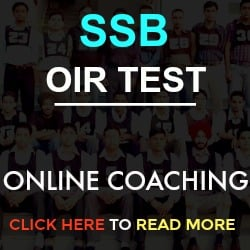## Must Know Cube And Dice MCQs For AFSB Interview OIR Test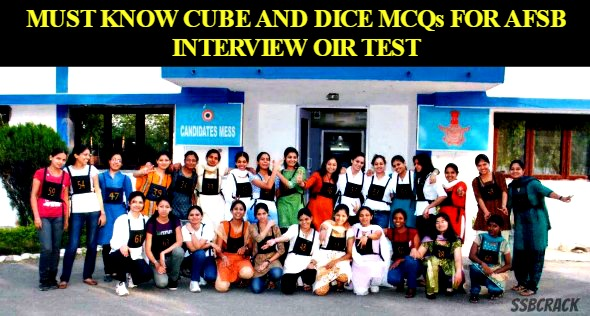In this article we will share SSB OIR Test 2016 Cubes and Dice Sample Questions, candidates must have a look to get an idea about the questions.
Directions to Solve

The sheet of paper shown in the figure (X) given on the left hand side, in each problem, is folded to form a box. Choose from amongst the alternatives (1), (2), (3) and (4), the boxes that are similar to the box that will be formed.

• Choose the box that is similar to the box formed from the given sheet of paper (X).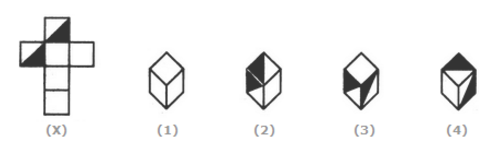A. 1 and 4 only
B. 3 and 4 only
C. 1 and 2 only
D. 2 and 3 only

• Choose the box that is similar to the box formed from the given sheet of paper (X).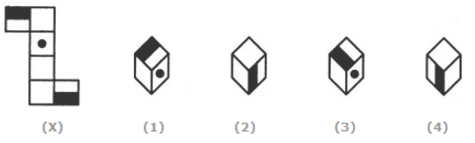A. 1 and 2 only
B. 2 and 3 only
C. 2 and 4 only
D. 1, 2, 3 and 4

• Choose the box that is similar to the box formed from the given sheet of paper (X).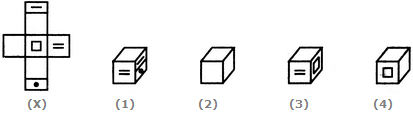A. 1 only
B. 1 and 3 only
C. 1, 3 and 4 only
D. 1, 2, 3 and 4

• Choose the box that is similar to the box formed from the given sheet of paper (X).A. 1 only
B. 2 only
C. 3 only
D. 4 only

• Choose the box that is similar to the box formed from the given sheet of paper (X).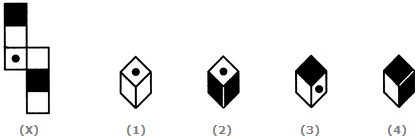A. 2 and 3 only
B. 1, 3 and 4 only
C. 2 and 4 only
D. 1 and 4 only

• Choose the box that is similar to the box formed from the given sheet of paper (X).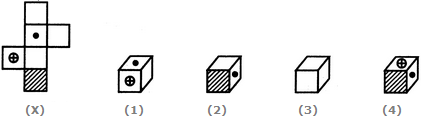A. 1 only
B. 1 and 3 only
C. 1, 3 and 4 only
D. 1, 2, 3 and 4

• Observe the dots on a dice (one to six dots) in the following figures. How many dots are contained on the face opposite to that containing four dots?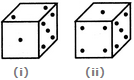A. 2
B. 3
C. 6
D. Cannot be determined

• Three positions of a dice are given. Based on them find out which number is found opposite the number 2 in the given cube.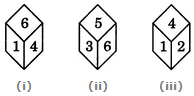A. 6

B. 5

C. 3

D. 1

• Two positions of a parallelepiped are shown below. When the number 3 will be on the top side, then which number will be at the bottom?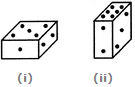A. 1

B. 4

C. 5

D. 6

• If the total number of dots on opposite faces of a cubical block is always 7, find the figure which is correct.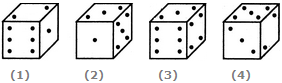A. Fig.1

B. Fig.2

C. Fig.3

D. Fig.4

• Two positions of a block are shown below: When six is at the bottom, what number will be at the top?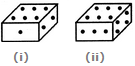A. 1

B. 2

C. 4

D. 5

• Four positions of a dice are shown below. What number must be at the bottom face when the dice is in the position as shown in the figure(iii)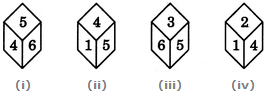A. 1

B. 2

C. 4

D. 6

• The four different positions of dice are given below: Which number is on the face opposite 6?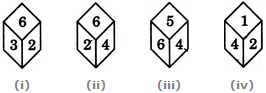A. 1

B. 2

C. 3

D. 4

• A cube has six different symbols drawn over its six faces. The symbols are dot, circle, triangle, square, cross and arrow. Three different positions of the cube are shown in figures X, Y, and Z. Which symbol is opposite the dot?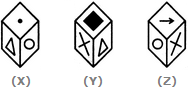A. Circle

B. Triangle

C. Arrow

D. Cross#### Ashutosh

Defence exams mentor, cracked CDS and AFCAT exams.

View all Posts

#### 1 Comment

1.srividya